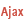Home |Sign in | EnglishHelpWLanguageWLanguage functionsStandard functionsFunctions for managing numeric valuesMathematical functionsAbsPresentationExampleDecimal separatorPrecision of the realsSee alsoAbsArcCosArcSinArcTan2ArcTanCoTanConversionCosDecimalPartDecimalToSexagesimalExpFactorialIntegerPartIsEvenIsOddLnLogMathematical functionsMaxMeanMinPowerRootRoundRoundDownRoundUpSexagesimalToDecimalSinSumTangent
 Decimal separatorPrecision of the reals WINDEVWEBDEVWINDEV MobileOthersAbs (Function) In french: Abs Returns the absolute value of a numeric value or numeric expression (integer or real). Example // Retrieve the absolute valueResAbsoluteValue = Abs(-16.23) // Returns 16.23ResAbsoluteValue = Abs(4.51 + 16.23) // Returns 20.74 Syntax = Abs() : Integer or realSought absolute value. : Numeric expression, integer or realNumeric expression or numeric value to use. Remarks Decimal separatorThe decimal separator for the real numbers is the one defined in the control panel of Windows.Precision of the realsIf the parameter passed to the function is a real, the result is always an 8-byte real.If the parameter passed to the function is a 4-byte real, the result will be considered as being an 8-byte real, which may result in additional decimal places when displaying the number. For example: rValue is 4-byte real = -1.70Trace(Abs(rValue)) // Displays 1.700000047684 To avoid this type of problem, we advise you to use reals (without specifying the size) or currencies.Component : wd250mat.dll See also Minimum version required Version 9 This page is also available for…